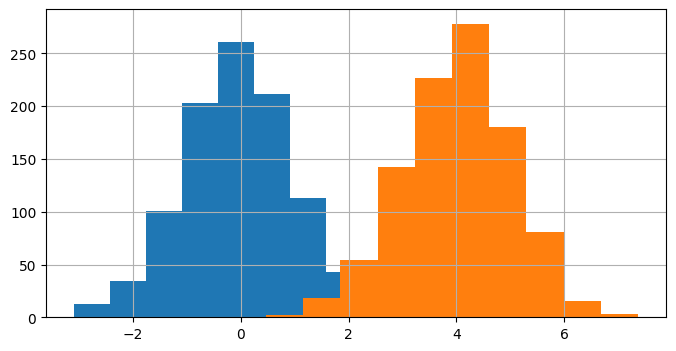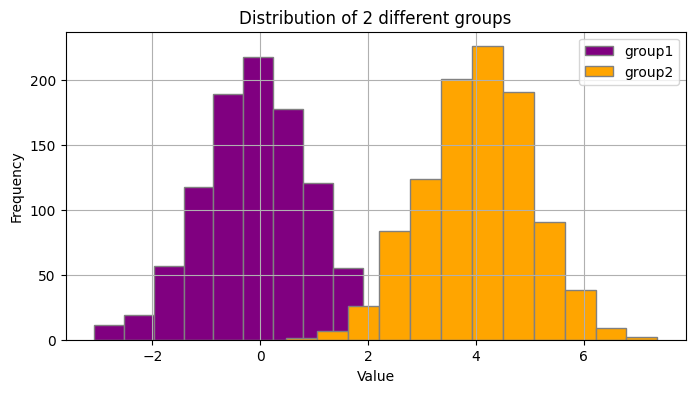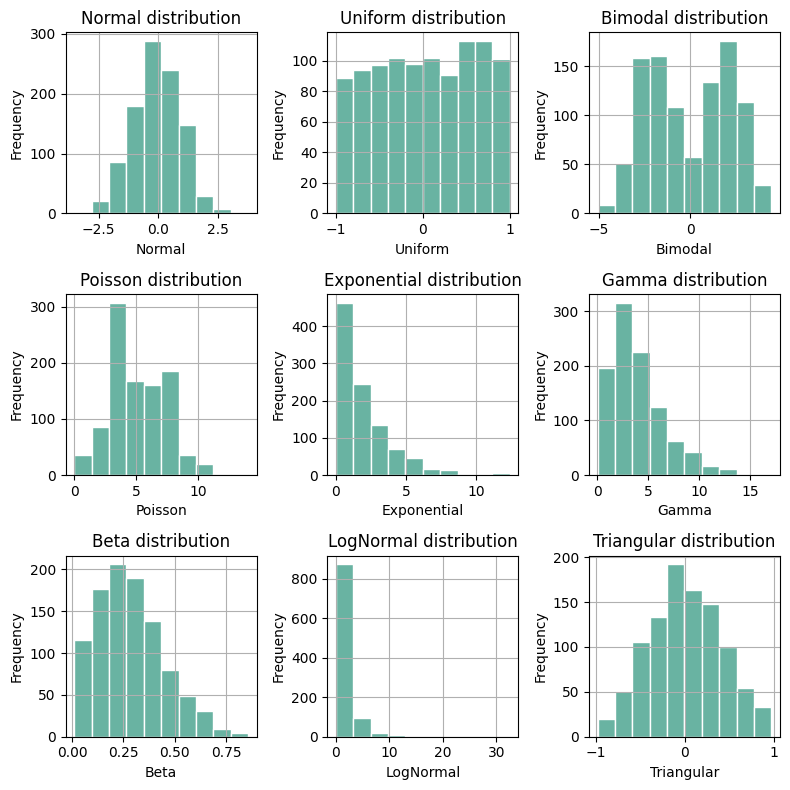## Libraries

Pandas is a popular open-source Python library used for data manipulation and analysis. It provides data structures and functions that make working with structured data, such as tabular data (like `Excel` spreadsheets or `SQL` tables), easy and intuitive.

To install Pandas, you can use the following command in your command-line interface (such as `Terminal` or `Command Prompt`):

`pip install pandas`

Matplotlib functionalities have been integrated into the pandas library, facilitating their use with `dataframes` and `series`. For this reason, you might also need to import the matplotlib library when building charts with Pandas.

This also means that they use the same functions, and if you already know Matplotlib, you'll have no trouble learning plots with Pandas.

``````import pandas as pd
import numpy as np
import matplotlib.pyplot as plt``````

## Dataset

In order to create graphics with Pandas, we need to use pandas objects: `Dataframes` and `Series`. A dataframe can be seen as an `Excel` table, and a series as a `column` in that table. This means that we must systematically convert our data into a format used by pandas.

Since histograms need quantitative variables, we will create a dataset with 2 columns. The first column is called `"type"`, which stores the categories `"group1"` and `"group2"` repeated a total of 1000 times each.

The second column is named `"value"`. It holds numbers. The first 1000 numbers are random values from a normal distribution with an average of 0 and a standard deviation of 1. The next 1000 numbers are random values from another normal distribution with an average of 4 and a standard deviation of 1. We concatenate them into one single column thanks to the `concatenate()` function from numpy.

``````# Create 2 columns: one categorical and one numerical
sample_size = 1000
data = {
'type': ['group1'] * sample_size + ['group2'] * sample_size,
'value': np.concatenate([np.random.normal(0, 1, sample_size),
np.random.normal(4, 1, sample_size)])
}
df = pd.DataFrame(data)``````

## Basic histogram with 2 groups

Once we've opened our dataset, we'll now create a simple histogram, representing the distributions of the `'value'` variable with the 2 groups. We will iterate over all distinct value in the `'type'` variable and use the `hist()` function.

``````# Plot the histograms of each group
for group in df['type'].unique():

# Filter the dataset on the group
filtered_df = df[df['type']==group]

# Add the histogram to the graphic
filtered_df['value'].hist(figsize=(8, 4))

# Display the plot
plt.show()``````## Customize histogram with 2 groups

The above histograms can be easily customized with the following features

• change the `bins` argument to the value we want
• change the `color` argument to the color we want
• change the `edgecolor` argument to the color we want
• add a title and axis label

Our first step will be to get a list of the labels in the `type` variable and then define a list of colors of the same length as the first list.

``````# Get group names and define colors
group_name = df['type'].unique()
colors = ['purple', 'orange']

# Plot the histograms
for i, group in enumerate(group_name):
ax = df[df['type']==group]['value'].hist(figsize=(8, 4),
edgecolor='gray',
bins=12,
color=colors[i]
)

ax.legend(group_name)

# Add a title and axis label
ax.set_title('Distribution of 2 different groups')
ax.set_xlabel('Value')
ax.set_ylabel('Frequency')

# Show the plot
plt.show()``````## Histogram with small multiples

Now we will see how to create a chart with small multiple histograms that display the distribution of several variables at the same time. First we need a dataset with more variables with different distributions.

### Create the dataset

For this, we will use `numpy` random functions and generate 9 different numeric variables. Don't worry if this seems complicated to you: it's only useful for generating fake data and making the graphs readable!

``````# Number of data points
num_data_points = 1000

# Generate data for each distribution
normal_data = np.random.normal(loc=0, scale=1, size=num_data_points)
uniform_data = np.random.uniform(low=-1, high=1, size=num_data_points)
bimodal_data = np.concatenate((np.random.normal(loc=-2, scale=1, size=num_data_points // 2),
np.random.normal(loc=2, scale=1, size=num_data_points // 2)))
poisson_data = np.random.poisson(lam=5, size=num_data_points)
exponential_data = np.random.exponential(scale=2, size=num_data_points)
gamma_data = np.random.gamma(shape=2, scale=2, size=num_data_points)
beta_data = np.random.beta(a=2, b=5, size=num_data_points)
lognormal_data = np.random.lognormal(mean=0, sigma=1, size=num_data_points)
triangular_data = np.random.triangular(left=-1, mode=0, right=1, size=num_data_points)

# Create a DataFrame
data = {
'Normal': normal_data,
'Uniform': uniform_data,
'Bimodal': bimodal_data,
'Poisson': poisson_data,
'Exponential': exponential_data,
'Gamma': gamma_data,
'Beta': beta_data,
'LogNormal': lognormal_data,
'Triangular': triangular_data
}

df = pd.DataFrame(data)``````

### Create the chart

Now we can create a small multiple histograms with pandas and matplotlib:

• The following code goes through each column of the dataframe and creates a histogram plot
• For each subplot, the code adds a histogram of a specific column's data from the dataframe
• It adds a title and axis label
• The code adjusts the layout (thanks to the `tight_layout()` function) to make sure they fit well together in the figure
• Finally, it displays the entire set of subplots as a single plot
``````# Initialize a 3x3 charts
fig, axes = plt.subplots(nrows=3, ncols=3, figsize=(8, 8))

# Flatten the axes array (makes it easier to iterate over)
axes = axes.flatten()

# Loop through each column and plot a histogram
for i, column in enumerate(df.columns):

df[column].hist(ax=axes[i], # Define on which ax we're working on
edgecolor='white', # Color of the border
color='#69b3a2' # Color of the bins
)

# Add title and axis label
axes[i].set_title(f'{column} distribution')
axes[i].set_xlabel(column)
axes[i].set_ylabel('Frequency')

plt.tight_layout()

# Show the plot
plt.show()``````## Going further

This post explains how to show the distribution of multiple groups and variables with pandas.

For more examples of how to create or customize your plots with Pandas, see the pandas section. You may also be interested in how to customize your histograms with Matplotlib and Seaborn.

## Contact & Edit

👋 This document is a work by Yan Holtz. You can contribute on github, send me a feedback on twitter or subscribe to the newsletter to know when new examples are published! 🔥